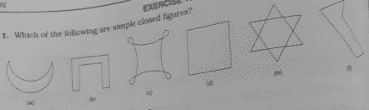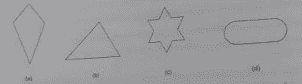RS Aggarwal Class 6 Solutions Chapter 15 - Polygons

RS Aggarwal Class 6 Chapter 15 - Polygons Solutions Free PDF

RS Aggarwal class 6 solutions chapter 15 polygons, is provided here. Solve questions based on polygons from RS Aggarwal for class 6. Solving questions from RS Aggarwal will help the students to understand the concepts of polygons in a better and easy way.

Exercise 15

Q1) Which of the following are simple closed figures?Soln:

1. It is a simple closed figure because it does not intersect itself.
2. It is a simple closed figure because it does not intersect itself.
3. It is not a simple closed figure because it intersects itself.
4. It is a simple closed figure because it does not intersect itself.
5. It is not a simple closed figure because it intersects itself.
6. It is a simple closed figure because it does not intersect itself.

Q2) Which of the following are polygon?Soln:

1. It is formed by four line segments and we know that a simple closed figure formed by three or more line segments is called a polygon. So it is a polygon named quadrilateral.
2. It is formed by three line segments and we know that a simple closed figure formed by three or more line segments is called a polygon. So it is a polygon named triangle.
3. It is formed by twelve line segments and we know that a simple closed figure formed by three or more line segments is called a polygon. So it is a polygon
4. It is not a polygon because it contains curves.

Q3) Fill in the blanks

(i) A polygon is a simple closed figure formed by more than _____ line segments.

(ii) A polygon formed by three line segments is called _____

(iii) A polygon formed by four line segments is called a _______

(iv) A triangle has ____ sides and _____ angles.

(v) A quadrilateral has ____ sides and ____ angles.

(vi) A figure which ends at the starting point is called a _______

Soln:

(i) A polygon is a simple closed figure formed by more than two line segments.

(ii) A polygon formed by three line segments is called triangle.

(iii) A polygon formed by four line segments is called a quadrilateral.

(iv) A triangle has three sides and three angles.

(v) A quadrilateral has four sides and four angles.

(vi) A figure which ends at the starting point is called a closed figure.

Practise This Question

Estimate the product of 958 × 387 by rounding off each factor to its greatest place.